# Smoothing Data Using Weighted Averages

Problem

You'd like to smooth data in a time series.

Solution

Use Excel's exponential smoothing feature in the Analysis ToolPak or construct a smoothed data series yourself using spreadsheet functions and VBA.

Discussion

Smoothing data is often desirable to remove unwanted noise in a data series. Computing moving averages as discussed in Recipe 6.3 is actually a smoothing process. Besides the moving averages methods discussed earlier, there are other ways to smooth data. Excel offers an exponential smoothing feature as part of the Analysis ToolPak. Further, you can construct any smoothing operations you desire using standard spreadsheet functions (and/or VBA). You already saw this approach in the previous recipe; however, this time I'll show you how to use a weighted moving average that makes use of a cubic spline interpolation kernel.

Exponential smoothing

Exponential smoothing is also a weighted-averaging technique. The idea behind weighted averaging is to give data values closest to the value being forecast or estimated greater importance, or influence, as opposed to values further away. Exponential smoothing uses the following formula: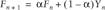Fn+1 is the value being estimated at time interval n + 1. a is a weighting factor, which is called a damping factor in Excel. Fn is the previous estimated value, and Yn is the previous actual value in the original data series.

To use exponential smoothing, select Tools [Image_Link]/images/U2192.jpg border=0> Data Analysis... from the main menu bar to open the Data Analysis dialog box (shown earlier in Figure 6-7). Select Exponential Smoothing from the list of available analyses. Doing so opens the Exponential Smoothing dialog box shown in Figure 6-11.

Figure 6-11. Exponential Smoothing dialog box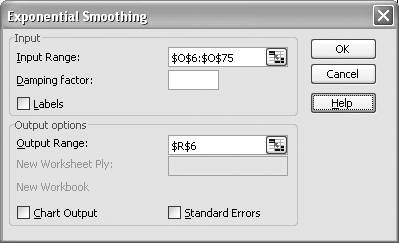In the Input Range field, type (or select on the spreadsheet) the cell range containing the input data you wish to smooth. Enter a damping factor in the "Damping factor" field or leave it blank to use the default value of 0.3. Enter a reference to the topmost cell in the desired output cell range in the Output Range field.

Press OK when you're done and you should see the results on your spreadsheet. Figure 6-12 shows an example for the same annual temperature data used in the previous recipe. The resulting data series after smoothing is contained in column R.

Figure 6-12. Exponential smoothing results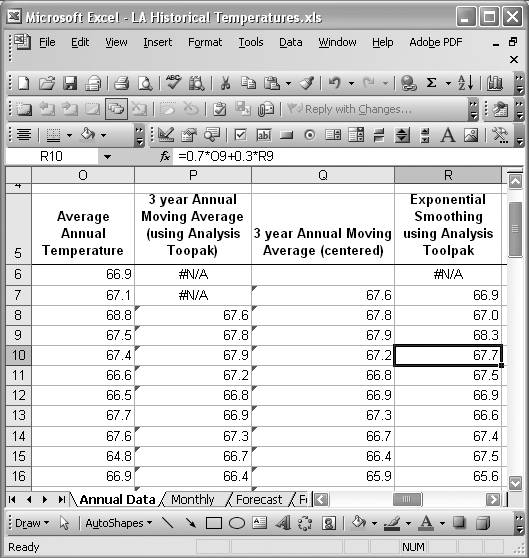Just like the Moving Average tool discussed earlier, the Exponential Smoothing tool places a cell formula rather than a value in each cell of the resulting data series. This way, if your original data changes, the smoothed data will update automatically. Cell R10 is selected in Figure 6-12 so you can see what the formulas look like. The formula is shown in the formula bar and is of the form =0.7*O9+0.3*R9. As you can see, this formula corresponds to the exponential smoothing equation I showed you earlier. Of course, you can enter these formulas yourself, bypassing the need to use the Analysis ToolPak, if you desire.

Figure 6-13 shows a plot of the smoothed data series superimposed over the original series so you can see the difference between the two.

Figure 6-13. Exponentially smoothed temperature data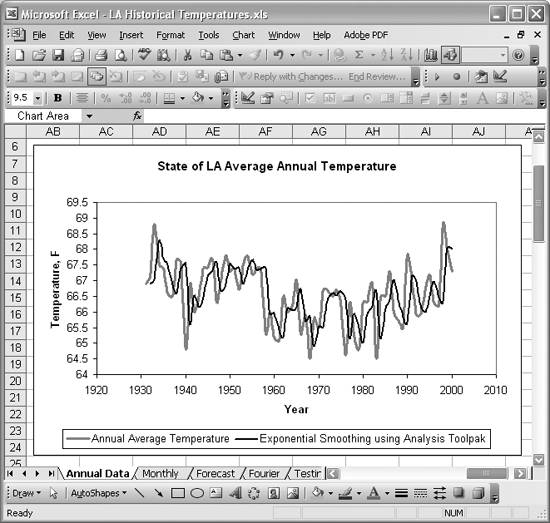Other weighted averaging techniques are commonly used in time series analysis. In the next section I'll show you one based on a cubic spline interpolation kernel.

Kernel smoothing

In this example, I'll show you a weighted averaging technique that uses a cubic spline interpolation kernel designed to approximate a Gaussian kernel. You can use all sorts of different kernels for smoothing with only slight modifications to the technique I'll present. The kernel I'll use is: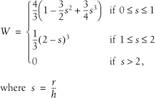r represents the distance between data points and h represents the effective smoothing radius divided by 2. I'll use this smoothing function to compute weights when computing weighted averages of the time series. To make this task easier, I added a VBA function called Wcs that you call from the spreadsheet. Example 6-1 shows my VBA implementation for this smoothing kernel.

Example 6-1. Cubic spline kernel

 ```Public Function Wcs(r As Double, h As Double) As Double Dim s As Double Dim w As Double   s = r / h   If s > 2 Then w = 0 ElseIf (s <= 2) And (s > 1) Then w = (1# / 3#) * (2# - s) ^ 3 ElseIf (s <= 1) And (s >= 0) Then w = (4# / 3#) * (1 - (3# / 2#) * s ^ 2 + (3# / 4#) * s ^ 3) End If   Wcs = w End Function ```

This is a fairly straightforward implementation of the equation I showed earlier, so I won't go over each line of code. Refer to Chapter 2 if you're not already up to speed on using VBA and adding custom functions and subroutines.

I'll use this function to generate weights in order to smooth the same temperature data discussed earlier. Figure 6-14 shows a weight table I computed using this function.

Figure 6-14. Weight table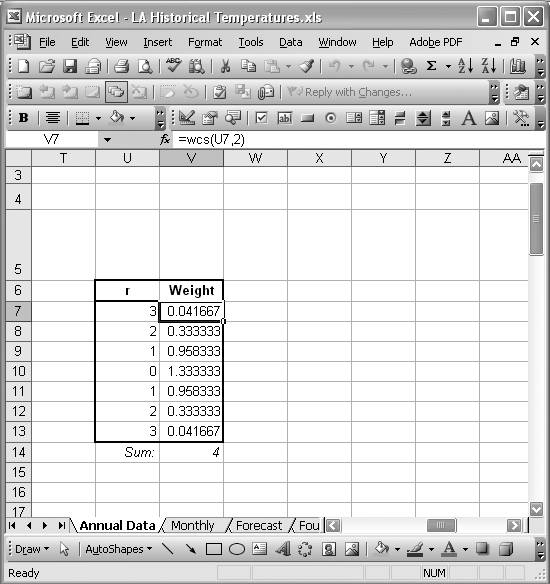The first column in the table, labeled r, contains relative indices around the fourth index, labeled 0. We can do this here to compute r because the time series data is sampled at evenly spaced intervals. The second column contains formulas like =Wcs(U10,2). This is the formula contained in cell V10. All the other cells contain similar formulas. The sum of the weights (computed using the SUM formula shown in cell V14) is required to normalize the weighted average computation. You can avoid this step if your kernel integrates to unity over its range of support.

Now, to compute the smoothed data series, I set up another column in my spreadsheet, as shown in Figure 6-15.

Figure 6-15. Kernel-smoothing results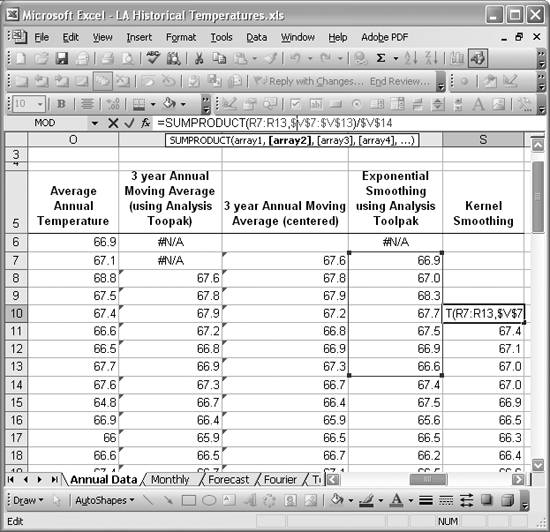The smoothed data is contained in column S under the heading Kernel Smoothing. I selected the first smoothed data value in cell S10 in the figure so you can see the cell formula. The formula is =SUMPRODUCT(R7:R13,\$V\$7:\$V\$13)/\$V\$14. As you can see, it uses the SUMPRODUCT formula to compute the sum of the products of each corresponding term in the two given cell ranges (see Chapter 7 for more information on this and other handy functions). In this case, the first cell range corresponds to the six data items around the given data item, including the given data item. This means the weighted average in this case is centered over the ith data item and includes the weighted influence of the three previous items and the three following items.

The second cell range contains the weights shown in Figure 6-14. Further, dividing by the sum of weights, contained in cell V14, normalizes the result. Once the first formula was set up, I simply copied and pasted it into the remaining cells in the data series.

Notice that we do lose some data. Specifically, we lose the first and last three data items in the range because of the range of influence of the weighting function I used. You can increase the range of influence by increasing the value for the h parameter passed into Wcs, or you can decrease it by decreasing this value. The greater the range of influence (hence the greater the number of data items in the series averaged for each smoothed result), the smoother the resulting data series.

Figure 6-16 shows a plot of the smoothed data series compared to the original data series; the effect of smoothing is clearly evident.

Figure 6-16. Kernel-smoothed temperature data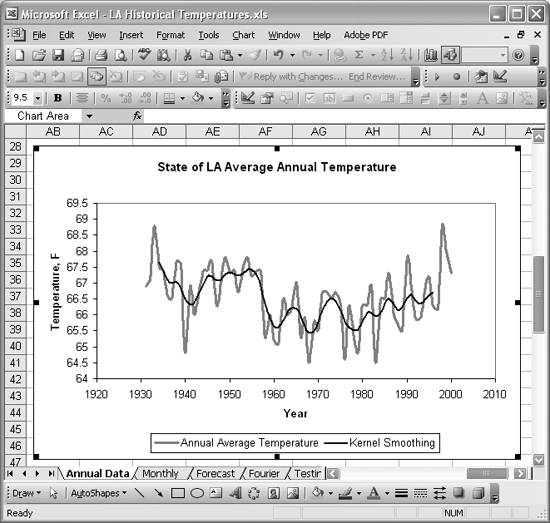See Recipe 6.3 to learn how to compute non-weighted moving averages in Excel. Smoothing data using the techniques in this and the previous recipe is actually a form of filtering. You can also filter data in the frequency domain in Excel using Fourier transforms; read Recipe 6.10 to learn more.Excel Scientific and Engineering Cookbook (Cookbooks (OReilly))
ISBN: 0596008791
EAN: 2147483647
Year: N/A
Pages: 206
Authors: David M Bourg